# Possible Triangle Constructions Projector Resources Possible Triangle Constructions

• Slides: 13
Download presentation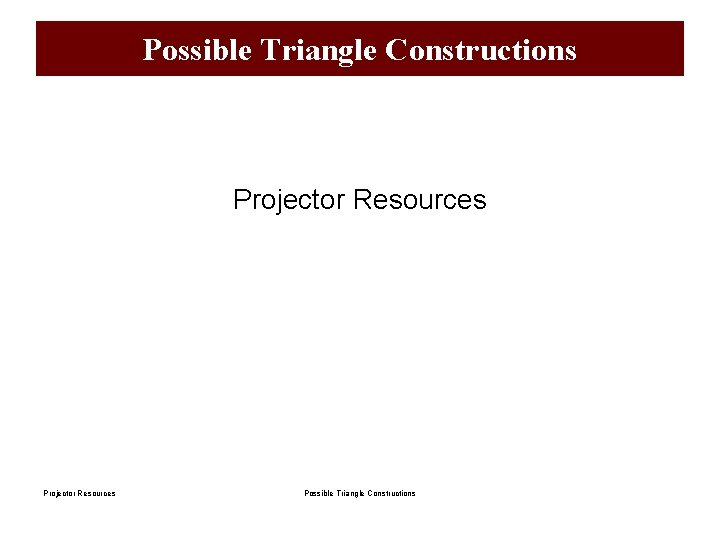Possible Triangle Constructions Projector Resources Possible Triangle Constructions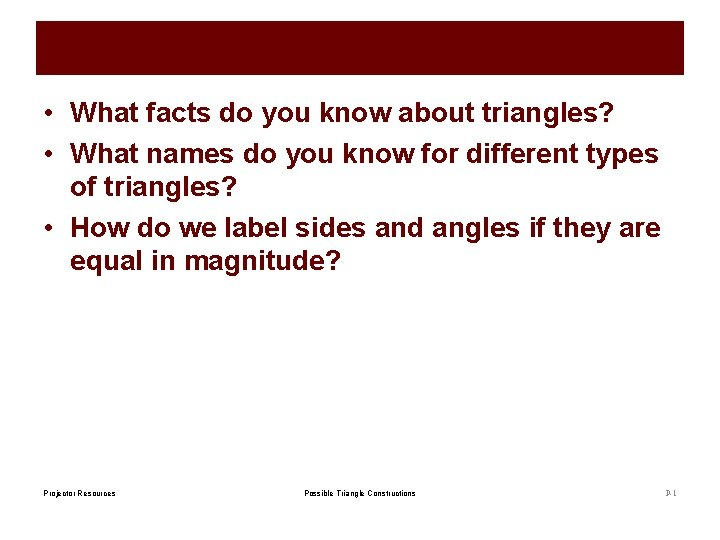• What facts do you know about triangles? • What names do you know for different types of triangles? • How do we label sides and angles if they are equal in magnitude? Projector Resources Possible Triangle Constructions P-1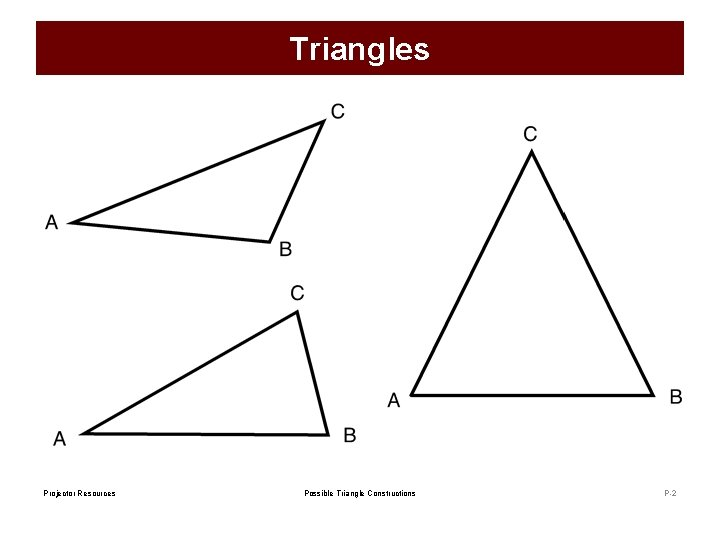Triangles Projector Resources Possible Triangle Constructions P-2• The sum of the interior angles of all triangles is 180 ⁰. • Triangles can be equilateral, isosceles, scalene, right angled, acute, obtuse… … Projector Resources Possible Triangle Constructions P-3Possible Triangle ABC? We know the length of two sides and one angle. Is it possible to construct a triangle with these properties? If yes, please create a sketch. If no, please explain why. AB = 4 cm, AC = 4 cm, Angle B = 40°. Projector Resources Possible Triangle Constructions P-4• Use mathematical reasoning to determine whether or not the information given describes a possible triangle. • Think carefully about any sketches you may draw and what your sketch can tell you about a possible triangle construction. Projector Resources Possible Triangle Constructions P-5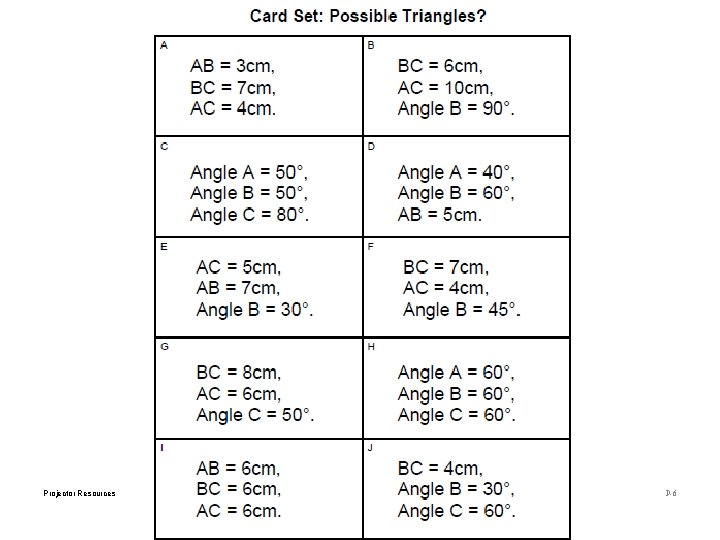Projector Resources Possible Triangle Constructions P-6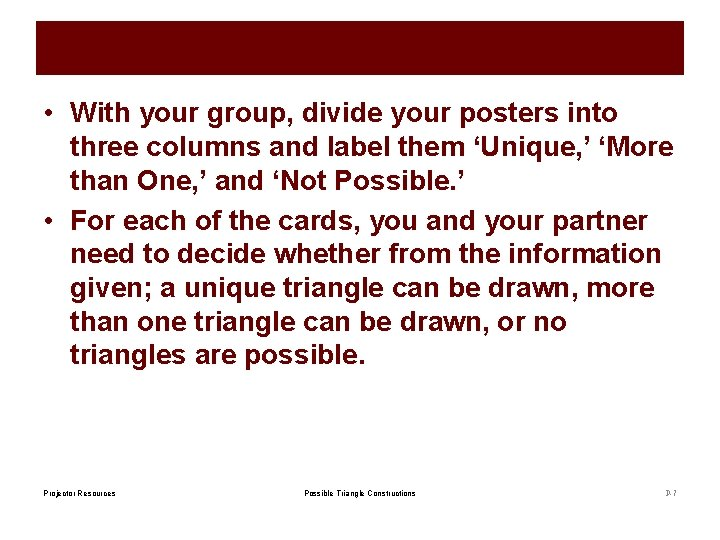• With your group, divide your posters into three columns and label them ‘Unique, ’ ‘More than One, ’ and ‘Not Possible. ’ • For each of the cards, you and your partner need to decide whether from the information given; a unique triangle can be drawn, more than one triangle can be drawn, or no triangles are possible. Projector Resources Possible Triangle Constructions P-7Working Together 1. Take turns to select any card. 2. Work individually: can a triangle be produced? 3. Decide together how many triangles are possible (one, more than one, none). • If a triangle is not possible, agree on your reasoning. • If you disagree, challenge each other’s explanations and work together to resolve your disagreements. 4. Once agreed, glue the card in the appropriate column and write an explanation in pencil on your poster, before moving on to another card. Projector Resources Possible Triangle Constructions P-8Think About… … • How do the given side lengths relate to each other? • Is there any information you have not been given that you need or could work out? What does this tell you about the number of possible triangles? • Why can you draw more than one triangle for one card, but not another card? Projector Resources Possible Triangle Constructions P-9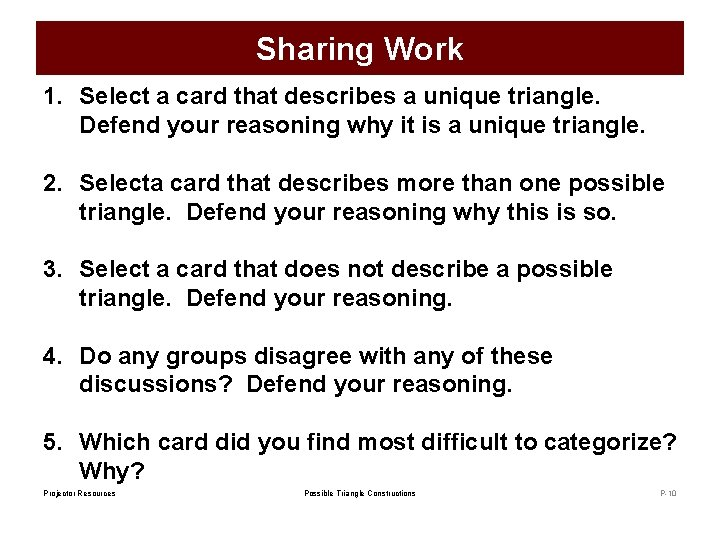Sharing Work 1. Select a card that describes a unique triangle. Defend your reasoning why it is a unique triangle. 2. Selecta card that describes more than one possible triangle. Defend your reasoning why this is so. 3. Select a card that does not describe a possible triangle. Defend your reasoning. 4. Do any groups disagree with any of these discussions? Defend your reasoning. 5. Which card did you find most difficult to categorize? Why? Projector Resources Possible Triangle Constructions P-10Choose Some Measures • Suggest some measurements for angles A and B that would make triangle ABC a possible triangle. • How many possible triangles do these measurements make? • Can you suggest measurements that would make the triangle unique? How about impossible? Angle A = …. . , Angle B = …. . , Angle C = 50°. Projector Resources Possible Triangle Constructions P-11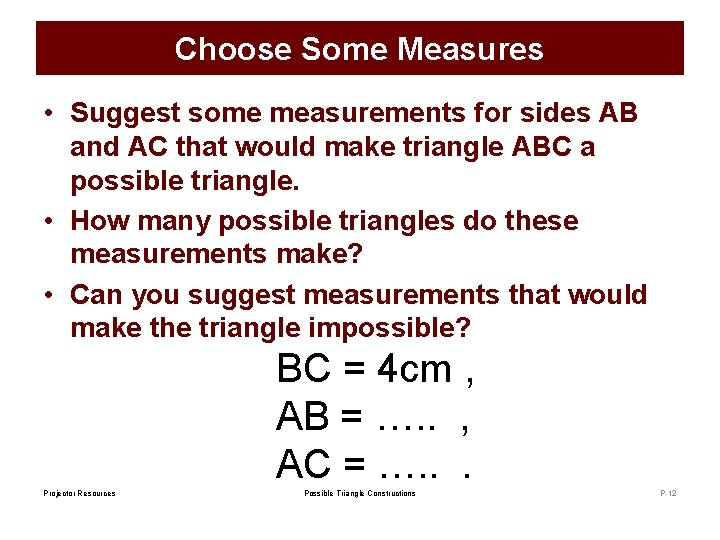Choose Some Measures • Suggest some measurements for sides AB and AC that would make triangle ABC a possible triangle. • How many possible triangles do these measurements make? • Can you suggest measurements that would make the triangle impossible? BC = 4 cm , AB = …. . , AC = …. . . Projector Resources Possible Triangle Constructions P-12